>

## Solutions of Reflection of Light Lakhmir Singh Manjit Kaur VSAQ, SAQ, MCQ, HOTS, and LAQ Pg No. 173 Class 10 Physics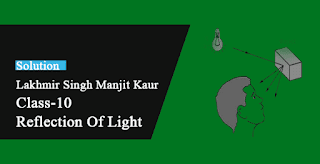Very Short Answer Type Questions-Pg-173

1. What happens when a ray of light falls normally (or perpendicular) on the surface of a plane mirror?

→ When a ray of light falls normally (or perpendicular) on the surface of a plane mirror, it means that angle of incidence is 0o. According to second law of reflection, angle of incidence is equal to the angle of reflection. Therefore, angle of reflection will also be zero. Thus, the light ray will be reflected back along the same path.

2. A ray of light is incident normally on a plane mirror at an angle 30°. What is the angle of reflection?

→ Second law of reflection says that angle of incidence is equal to the angle of reflection.
Angle of incidence =30°
Thus, angle of reflection=30°

3. A ray of light strikes a plane mirror at an angle of 40° to the mirror surface. What will be the reflection?

→ Angle made by the incident ray with respect to mirror surface = 40°
Therefore, angle of incidence= 90° - 40° = 50°
Second law of reflection says that angle of incidence is equal to the angle of reflection.
Therefore, angle of reflection= angle of incidence = 50°

4. A ray of light is incident normally on a plane mirror. What will be the:
(a) Angle of incidence?
(b) Angle of reflection?

→ (a) Angle of incidence is 0°
(b) Angle of reflection is 0°

5. What type of image is formed?
(a) In a plane mirror?
(b) On a cinema screen?

→ (A) In a plane mirror image formed is Virtual image
(b) On a cinema screen image formed is Real image

6. What kind of mirror is required for obtaining a virtual image of the same size as the object?

→ Plane mirror is required for obtaining a virtual image of the same size as the object.

7. What is the name of the phenomenon in which the right side of an object appears to be the left side of the image in a plane mirror?

→ The name of the phenomenon in which the right side of an object appears to be the left side of the image in a plane mirror is Lateral inversion.

8. Name the phenomenon responsible for the following effect:
When we sit in front of a plane mirror and write with our right hand, it appears in the mirror that we are writing with the left hand.

→ The phenomenon responsible for the effect that when we sit in front of a plane mirror and write with our right hand, it appears in the mirror that we are writing with the left hand is Lateral inversion.

9. If an object is placed at a distance of 10 cm in from of a plane mirror, how far would it be from it’s from?

→ Since, when the object is kept in front of the mirror then the image is formed behind the mirror at the same distance as the object distance from the mirror.
Object and mirror distance =10 cm
Mirror and image distance =10 cm
Therefore, the distance between image and object =10+10=20cm.

10. Which property of light makes a pencil cast a shadow when it is held in front of a light source?

→ The property of light makes a pencil cast a shadow when it is held in front of a light source is that light travels in a straight line.

11. The image seen in a plane mirror cannot be formed on a screen. What name is given to this type of image?

→ The name given to this type of image is Virtual image.

12. Fill in the following blanks with a suitable word:
When light is reflected, the angles of reflections and incidence are _______

→ Equal.

13. State whether the following statement is true or false:
A student says that an object can be seen because light is reflected back by the object to our eyes.

→ False.

14. Where is the image when you look at something in a mirror?

→ The image is formed behind the mirror when we look at something in a mirror.

15. A ray of light strikes a plane mirror such that its angle of incidence is 30°. What angle the reflected ray make with the mirror surface?

→ Second law of reflection says, angle of incidence is equal to the angle of reflection.
Angle of incidence=30°
Such that angle of reflection=30°
Now, the angle made by the reflected ray with mirror surface=90°-30°=60°.

Short Answer Type Questions-Pg-173

16. What is the difference between a real image and a virtual image? Give one example of each type of image.

→ Since light rays pass through a real image thus it can be obtained on a screen like image formed on a cinema screen whereas light rays do not actually pass through a virtual image thus it cannot be formed on screen like image formed by a plane mirror.

17. The letter E is placed in front of a plane mirror:
(a) How would its image look like when seen in a plane mirror?
(b) What is the name of the phenomenon involved?

→ (a) Image of E will look like when seen in a plane mirror as.
(b) The name of the phenomenon involved is lateral inversion.

18. What is lateral inversion? Explain by giving a suitable example.

→ When an object is placed in front of a plane mirror, then the left side of the object appears to become right side of the image; and the right side of the object appears to become the left side of the image.
The change of the sides of an object and its mirror image is called lateral inversion.
Example. When we hold our hand in front of a mirror the thumb on right appears to be on left.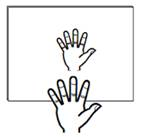19. Write the word AMBULANCE as it would appear when reflected in a plane mirror. Why is it sometimes written in this way (as its mirror image) on the front of an ambulance?

→ The word AMBULANCE it would appear like this when reflected in a plane mirror.
It is sometimes written in this way on the front of an ambulance so that while driving, if we see in our rear-view mirror that a hospital van is coming from behind, then we will get the laterally inverted image of  and read it as AMBULANCE and give way for it to pass through.

20. What are the important difference between looking at a photograph of your face and looking at yourself in a plane mirror?

→ The important difference between looking at a photograph of our face and looking at ourselves in a plane mirror is that the image of our face in a plane mirror is laterally inverted, so right is left and left is right. However, in a photograph of our face this does not happen.

21A. A wall reflects light and a mirror also reflects light. What difference is there in the way they reflect light?

→ The difference between reflection of light from wall and mirror is that the reflection from wall is a diffuse reflection as it has rough surfaces. A parallel beam of light incident on it is reflected in different directions.
Whereas by a mirror it is a regular reflection as mirror surface is smooth. A parallel beam of light incident on it gets scattered by making reflected rays in different directions.

21B. Which type of reflection of light leads to the formations of images?

→ Regular reflection is the type of reflection of light that leads to the formations of images.

22. What is the difference between regular reflection of light and diffuse reflection of light? What type of reflection of light takes place from?
(a) A cinema screen
(b) A plane mirror
(c) A cardboard
(d) Still water surface of a lake

→ The difference between regular reflection of light and diffuse reflection of light is that in regular reflection, a parallel beam of incident light is reflected as a parallel beam only in one direction; while in diffuse reflection, a parallel beam of incident light is reflected in different directions.
(a) The type of reflection of light that takes place from a cinema screen is Regular reflection
(b) The type of reflection of light that takes place from a plane mirror is Regular reflection
(c) The type of reflection of light that takes place from a cardboard is diffuse reflection
(d) The type of reflection of light that takes place from still water surface of a lake is Regular reflection.

23. What can you see in a completely dark room? If you switch on an electric bulb in this dark room as a light source, explain how you could now see:
(a) The electric bulb
(B) a piece of white paper.

→ When we see in a completely dark room, we see nothing as there is any light in the dark room.
(a) If we switch on an electric bulb in this dark room as a light source we can see bulb due to the light emitted by it.
(b) If we switch on an electric bulb in this dark room as a light source we can see a piece of white paper as it reflects the light from the bulb falling on it.

24A. A boy with a mouth 5 cm wide stands 2 m away from a plane mirror. Where is his image and how wide is the image of his mouth ?

→ Height of mouth = 5cm
Mouth mirror distance = 2m
Second law of reflection says, angle of incidence is equal to the angle of reflection.
Thus, the image will form 2 m behind the mirror and the width of the image of boy's mouth will be 5 cm.

24B. The boy walks towards the mirror at a speed of 1m/s. At what speed does his image approach him?

→ When the boy walks towards the mirror at a speed of 1 m/s, his image will also appear to move towards the mirror at the same speed of 1 m/s. So, the speed at which his image approach him will be 1 m/s + 1 m/s = 2 m/s.

25A. An extended object in the form of an arrow pointing upwards has been placed in front of a plane mirror Draw a labeled ray-diagram to show the formation of its image.

→ An extended object in the form of an arrow pointing upwards has been placed in front of a plane mirror. Tithe labeled ray-diagram to show the formation of its image is25B. State the uses of plane mirrors.

→ Uses of Plane mirrors:
(I) Plane mirrors are used to see ourselves. Example mirror on our dressing table is plane mirror.
(ii) Inside the walls of certain shops to make them look bigger.
(iii) Plane mirrors are used to make periscopes.
(iv) At blind turns of some busy roads so that drivers can see the vehicles coming from the other side and prevent accidents.

Long Answer Type Questions-Pg-174

26. What is meant by ‘reflection of light’? Define the following terms used in the study of reflection of light by drawing a labeled ray-diagram:
(a) Incident ray
(b) Point of incidence
(c) Normal
(d) Reflected ray
(e) Angle of incidence
(f) Angle of reflection

→ The process of bouncing back off the light rays which fall on the surface of an object is called reflection of light.(a) Incident ray: This is the ray of light that falls on the mirror surface.
(b) Point of incidence: This is the point at which the incident ray falls on the mirror.
(c) Normal: The line at right angle to the mirror surface at the point of incidence is called normal.
(d) Reflected ray: The ray of light which is reflected back by the mirror is called the reflected rays.
(e) Angle of incidence: The angle made by the incident ray with the normal at the point of incidence is called angle of incidence.
(f) Angle of reflection: The angle made by the reflected ray with the normal at the point of incidence is called angle of reflection.

27. State and explain the laws of reflection of light at a plane surface (like a plane), with the help of a labeled ray-diagram. Mark the angles of ‘incidence’ and ‘reflection’ clearly on the diagram. If the angle of reflection is 47.5°, what will be the angle of incidence?

→ Laws of reflection of light states that:First law of reflection: The incidence ray, the reflected ray and the normal (at the point of incidence), all lie in the same plane.
For e.g. in the figure, the incident ray, the reflected ray and the normal, all lie in the same plane, the plane of paper.
Second law of reflection: The angle of reflection is always equal to the angle of incidence.
For e.g.
If angle of reflection is 45°, then the angle of incidence will also be 45°.

28. With the help of a labeled ray-diagram, describe how a plane mirror forms an image of a point source of light placed in front of it. State the characteristics of the image formed in a plane mirror.Characteristics of Image formed in plane mirror are:-1-Virtual Images are formed. 2- Image formed is upright. 3- Object Distance is same as Image Distance. 4-Size of the Image formed is same as size of the object.

29A. Explain why, though both a plane mirror and a sheet of paper reflect light but we can see the image of our face in a plane mirror but not in a sheet of paper.

→ Though both a plane mirror and a sheet of paper reflect light but we can see the image of our face in a plane mirror but not in a sheet of paper as images are formed by reflection of light which takes place in case of a plane mirror. In case of a sheet of paper, diffuse reflection takes place.

29B. The image in a plane mirror is virtual and laterally inverted. What does this statement mean?

→ The image is virtual and laterally inverted means that it cannot be obtained on a screen and is reversed sideways that is the right of the object is the left of the image and vice versa.

29C. Write all the capital letters of the alphabet which look the same in a plane mirror.

→ All the capital letters of the alphabet which look the same in a plane mirror are A, H, I, M, 0, T, U, V, W, X, Y.

Multiple Choice Questions (MCQs)-Pg-174

30. The angle of reflection is equal to the angle of incidence:
A. Never
B. Sometimes
C. Under special conditions
D. Always

→ Second law of reflection says that angle of incidence is equal to the angle of reflection.

31. The angle between an incident ray and the plane mirror is 30°. The total angle between the incident ray and reflected for this ray will be:
A. 30°
B. 60°
C. 120°
D. 90°32. A ray of light is incident on a plane mirror making an angle of 90° with the mirror surface. The angle of reflection for this ray of light will be:
A. 0°
B. 90°
C. 45°
D. 60°

→ Angle of incidence = 0°
Therefore, Angle of reflection = 0°.

33. The image of an object formed by a plane mirror is:
A. Real
B. virtual
C. diminished
D. upside-down

→ The image of an object formed by a plane mirror is virtual.

34. The image of an object formed by a plane mirror is:
A. Virtual, behind the mirror and enlarged.
B. Real, at the surface of the mirror and enlarged.
C. Virtual, behind the mirror and of the same size as the object.
D. Real, behind the mirror and of the same size as the object.

→ The image of an object formed by a plane mirror is Virtual, behind the mirror and of the same size as the object.

35. The figure given alongside shows the image of a clock as seen I a plane mirror. The correct time is: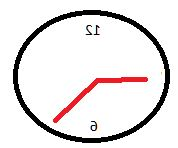A. 9.25
B. 2.35
C. 6.45
D. 2.25Questions Based on High Order Thinking Skills (HOTS)-Pg-175

36. A man stands 10 m in front of a large plane mirror. How far must he walk before he is 5 m away from his image?

→ Initially,
Man and the mirror distance= 10m.
Man and his image distance= 10+10=20m
Now, New distance between the man and his image= 5 m when the distance between man and mirror is 2.5 m.

Therefore, distance he has to walk towards the mirror = 10 m - 2.5m = 7.5 m.

37. An object is placed 20 cm in front of a plane mirror. The mirror is moved 2 cm towards the object. The distance between the positions of the original and final images seen in the mirror is:
A) 2 cm
B) 4 cm
C) 10 cm
D) 22 cm

→ Initially, object and mirror distance= 20 cm
Image and mirror distance = 20 cm
Now, mirror is moved 2 cm towards the object,
New distance between object and mirror = 20 cm - 2 cm = 18 cm
New distance between mirror and image = 20 cm - 2 cm = 18 cm,
It is 2 cm less than the distance in initial case.
The total distance between the positions of the original and final images is 2 cm + 2 cm = 4 cm since, the mirror has also moved 2 cm away from the position of original image.

38. A man sits in an optician's chair, looking into a plane mirror which is 2 m away from him and views the image of a chart which faces the mirror and is 50 cm behind his head. How far away from his eyes does the chart appear to be?

→ Man and the mirror distance= 2 cm
Man and the chart distance = 50 cm = 0.5 m
Chart and mirror distance = 0.5 m + 2 m = 2.5 m
Mirror and the image of the chart distance = 2.5 m
Now, man and the image of chart distance
= man and the mirror distance + mirror and the image of the chart distance
= 2 m + 2.5 m
= 4.5 m.

39. A ray of light strikes a plane mirror PQ at an angle of incidence of 30°, is reflected from the plane mirror and then strikes a second plane mirror QR placed at right angles to the first mirror. The angle of reflection at the second mirror is:
A) 30°
B) 45°
C) 60°
D) 90°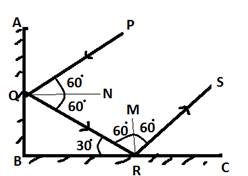Ray PQ strikes the mirror AB at Q and gets reflected along QR according to the laws of reflection. The ray QR incident on mirror BC makes an angle of 30° with the mirror. So, the angle of incidence on this mirror is 90° - 30° = 60°. Hence, the angle of reflection is also 60°.

40. Explain how to read the following message which was found on some blotting paper:

→ The impression on blotting paper is the mirror image of the written message;
THERE IS A MAN SITTING THERE.

Very Short Answer Type Questions-Pg-178

1. Name the spherical mirror which has:
(a) Virtual principal focus.
(b) Real principal focus.

→ (a) The spherical mirror which has virtual principal focus is convex mirror.

(b) The spherical mirror which has real principal focus is Concave mirror.

2. Out of convex mirror and concave mirror, whose focus is situated behind the mirror?

→ Focus of convex mirror is situated behind the mirror.

3. Find the focal length of a concave mirror whose radius of curvature is 30 cm.

→ Radius of curvature (R) = 30cm
Focal length (f) =?
We know that
f = R/2
=30/2
f = 15cm.

4. If the focal length of a convex mirror is 28 cm, what is its radius of curvature?

→ Focal length (f) = 28 cm
Radius of curvature (R) =?
We know that
f = R/2
28=R/2
R= 28 x 2
R = 56 cm.

5. Fill in the following blanks with suitable words:
(a) Parallel rays of light are reflected by a ______ to a point called the principal focus.
(b) The focal length of a concave mirror is the distance from the principal focus to the ____.
(c) A______ converges rays of light whereas a ______ diverges rays of light.
(d) For a convex mirror, parallel rays of light appear to ___ from a point called the principal focus.

→ (a) Concave mirror
(b) Mirror
(c) Concave mirror; convex mirror
(d) Diverge

Short Answer Type Questions-Pg-179

6. What is a spherical mirror? Distinguish between a concave mirror and a convex mirror.

→ Spherical mirror are those mirror whose reflecting surface is the part of a hollow sphere of glass. The two types of spherical mirrors are:
1) concave mirrors

2) convex mirrors7. Name the two types of spherical mirrors. What type of mirror is represented by the:
(a) Back side of a shining steel spoon?
(b) Front side of a shining steel spoon?

→ Two types of spherical mirrors are: Concave mirror and convex mirror
Type of mirror represented by the:
(a) Back side of a shining steel spoon is convex mirror
(b) Front side of a shining steel spoon is concave mirror

8. What is the relation between the focal length and radius of curvature of a spherical mirror (concave mirror or convex mirror)? Calculate the focal length of a spherical mirror whose radius of curvature is 26 cm.

→ For a spherical mirror the principal focus (F) lies exactly mid-way between the pole (P) and centre of curvature (C). So, the relation between focal length (f) of a spherical mirror and radius of curvature (R) is
f=R/2
Radius of curvature (R) = 26cm
Focal length (f) =?
We know that
f=R/2
=26/2
f =13cm.

9. Explain with a suitable diagram, how a concave mirror converges a parallel beam of light rays. Mark clearly the pole, focus and centre of curvature of concave mirror in this diagram.Since a concave mirror converges a parallel beam of light rays thus all the light rays parallel to the principal axis of a concave mirror, converge at the principal focus (F) after reflection from the mirror.

10. Describe with a suitable diagram, how a convex mirror diverges a parallel beam of light rays. Mark clearly the pole, focus and centre of curvature of convex mirror in this diagram.Since a convex mirror diverges a parallel beam of light rays thus all the light rays which are parallel to the principal axis of a convex mirror, appear to diverge from the principal focus (F) after reflection from the mirror.

Long Answer Type Questions-Pg-179

11. Define
(a) Center of curvature
(b) Radius of curvature
(c) Pole
(d) Principal axis, and
(e) Aperture, of a spherical mirror with the help of a labeled diagram.(a) Centre of curvature: center of the hollow sphere of glass of which the mirror is a part.
(b) Radius of curvature: radius of the hollow sphere of glass of which the mirror is a part.
(c) Pole: The centre of a spherical mirror is called its pole.
(d) Principal axis: The straight line passing through the centre of curvature and pole of a spherical mirror is called its principal axis.

(e) Aperture: The portion of a mirror from which the reflection of light actually takes place is called the aperture of the mirror.

12A. Define
(i) principal focus of a concave mirror, and
(ii) Focal length of a concave mirror.

→ (a) (i) the principal focus of a concave mirror is a point on its principal axis to which all the parallel light rays; (b) Converge after reflection from the concave mirror.
(ii) The focal length of a concave mirror is the distance between its pole and the principal focus.

12B. Draw diagram to represent the action of a concave mirror on a beam of parallel light rays. Mark on this diagram principal axis, focus F, center of curvature C, pole P and focal length f, of the Concave mirror.13A. What is meant by?
(i) principal focus of a convex mirror, and
(ii) Focal length of a convex mirror?

→ (i) Principal focus of a convex mirror: The principal focus of a convex mirror is a point on its principal axis from which all the parallel light rays; diverge after being reflected from the convex mirror.
(ii) The focal length of a convex mirror is the distance between its pole (P) and principal focus (F).

13B. Draw diagram to show the action of convex mirror on a beam of parallel light rays. Mark on this diagram principal axis, focus F, center of curvature C, pole P and focal length f, of the convex mirror.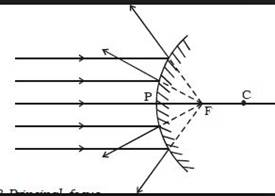Multiple Choice Questions (MCQs)-Pg-179

14. In a convex spherical mirror, reflection of light takes place at:
A. A bulging-out surface
B. A bent-in surface
C. A flat surface
D. An uneven surface

→ In a convex spherical mirror, reflection of light takes place at a bulging-out surface.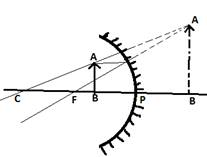15. A diverging mirrors:
A. A plane mirror
B. A concave mirror
C. A convex mirror
D. A shaving mirror

→ A convex mirror diverges the rays falling on it.

16. If R is the radius of curvature of a spherical mirror and f is its focal length, then:
A. R = f B. R = 3f
C. R = f D. R = 2f

→ F = R/2

17. The focal length of a spherical mirror of radius of curvature 38 cm is:
A. 10 cm
B. 19 cm
C. 20 cm
D. 30cm

→ F =R/2 = 38/2 = 19 cm.

18. If the focal length of a spherical mirror is 12.5 less cm, its radius of curvature will be:
A. 15 cm
B. 25cm
C. 20 cm
D. 35 cm

→ F = R/2
R = 2f = 2 x 12.5 cm = 25 cm.

Questions Based on High Order Thinking Skills (HOTS)-Pg-179

19. A communications satellite in orbit sends a parallel beam of signals down to earth. If these signals obey the same laws of reflection as light and are to be focused onto a small receiving aerial, what should be the best shape of the metal 'dish' used to collect them?

→ Concave metal dish: It will collect the parallel beam of satellite signals at its focus where receiving aerial is fixed.

20. When a spherical mirror is held towards the sun and its sharp image is formed on a piece of carbon paper for some time, a hole is burnt in the carbon paper.
(a) What is the nature of spherical mirror?
(b) Why is a hole burnt in the carbon paper?
(c) At which point of the spherical mirror the carbon paper is placed?
(d) What name is given to the distance between spherical mirror and carbon paper?
(e) What is the advantage of using a carbon paper rather than a white paper?